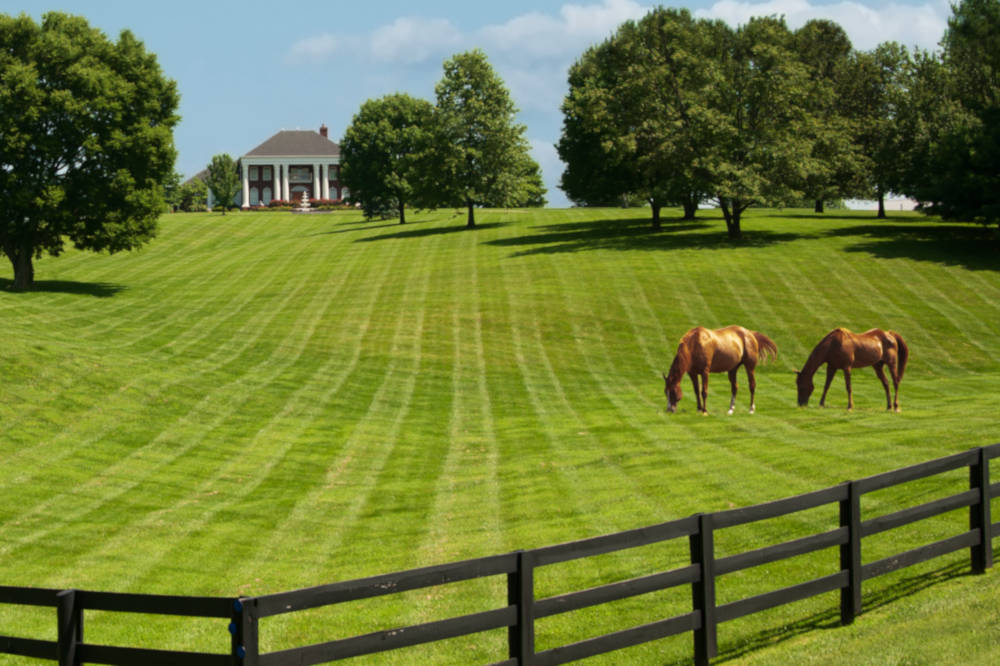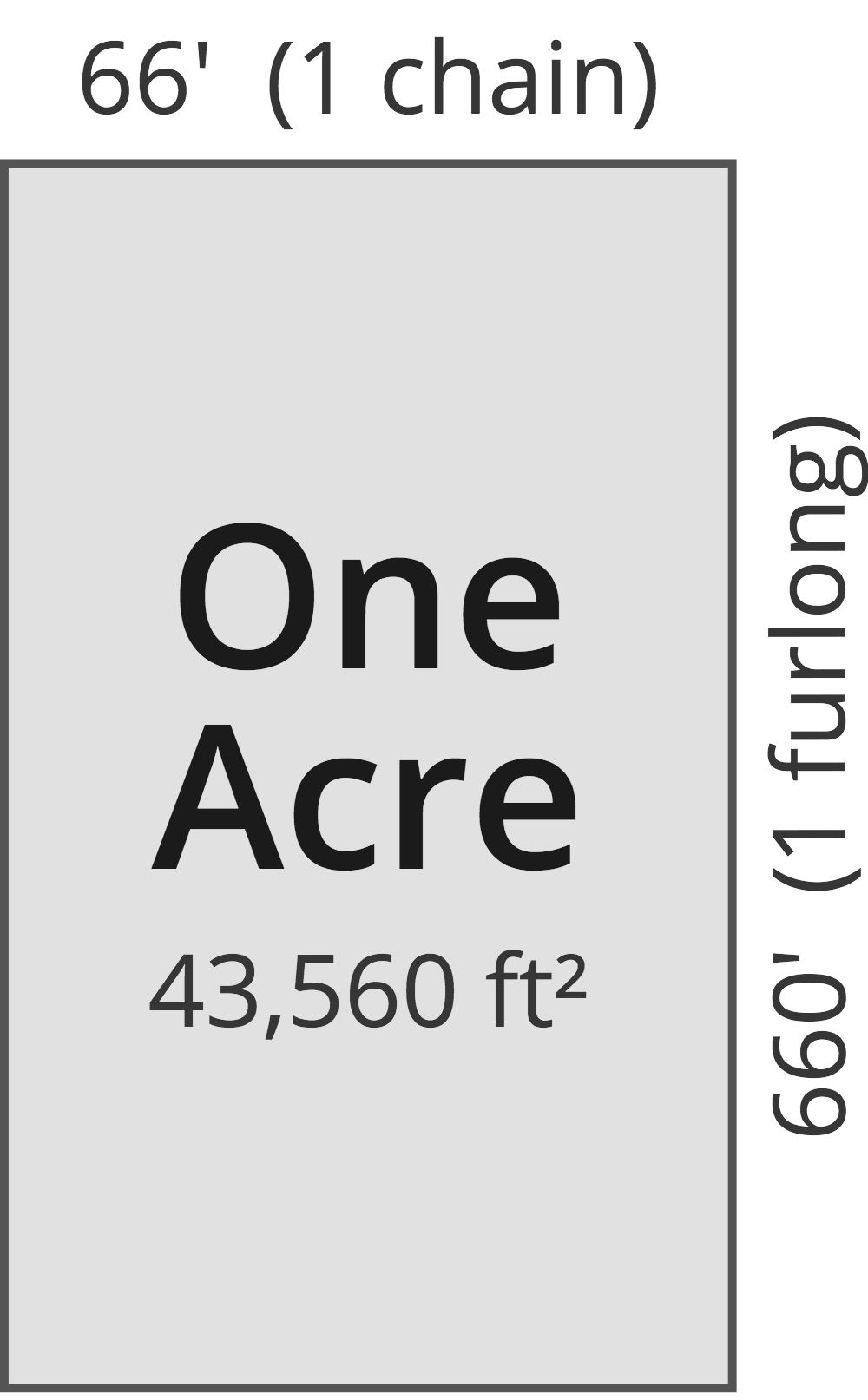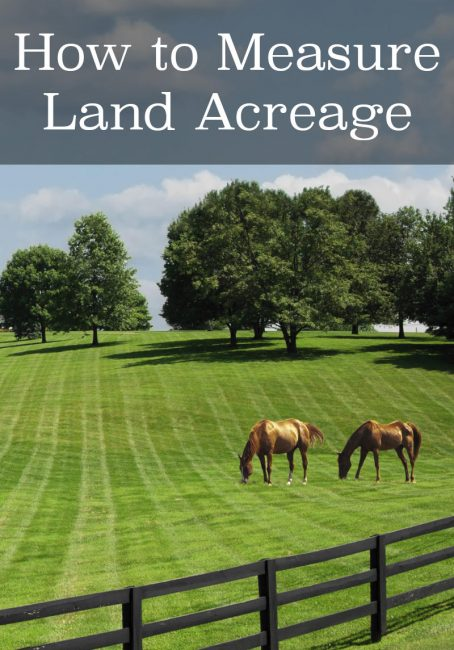# Acreage Calculator – Find Acres Using a Map or Land Dimensions

Calculate an area in acres by entering the length and width. For more complex shapes, use the map to calculate acreage by placing pins on the edges of the land to be measured.

## Total Acreage:

 Acres Hectares Square Feet Square Meters Square Miles Square Kilometers Perimeter in Feet Perimeter in Meters
Learn how we calculated this below

## What is an Acre

An acre is a measurement of land area used in the Imperial measurement system (U.S.A. and informally in the U.K. and its former colonies). It was originally the amount of land a yoke of oxen could plow in a day.

Now, it is defined as an area measuring one chain by one furlong — not entirely more useful units either!

We’ll discuss how much an acre is in a more reasonable way below. But, know that an acre is still a common land area measurement used in the U.S., especially in real estate and government land transactions.## How Big is an Acre

So having a dimension of one chain by one furlong, you might be wondering how big an acre is. One acre is equal to 43,560 square feet, which is just a little bit smaller than a football field and about the same size as a soccer field.

The dimensions of an acre have been historically defined as being equal to an area that is 66 feet (1 chain) by 660 feet (1 furlong).

Defining the size in acreage this way allowed farmers to more easily determine how much land they could plow per day. The furlong was originally the distance an oxen team could plow without rest. So they defined the acre in terms of how many furrows (the length of the furlong) could be plowed in a single day.Outside of land surveys, these units of measure are rarely used. Refer to the table below to see how big an acre is relative to more commonly used measurements.

### Size of One Acre

Here’s a quick reference table showing the size of an acre.

Chart showing the dimensions of one acre in various units of area measurement.
Unit of Measure Dimension Equal to 1 Acre
Hectares 0.4047 hectares
Meters 4,047 m²
Miles 0.0015625 miles² (640 acres = 1 mile²)
Yards 4,840 yds²
Square Feet 43,560 ft²
Square Inches 6,272,640 in²
Perches 160 perches (1 perch = 1 rod)
Rods 160 rod² (1 rod = 16 ½ feet)

## How to Calculate Acres

The easiest way to calculate the number of acres in your land is to use the calculator above. If you know the length and width, simply enter them. The calculator will provide the number of acres, as well as the area in other units.

It will also give you perimeter. If you already know the acres but want the area in other units, use our acreage unit conversion tool.

To calculate acres by hand, multiply your length and width (in feet) to get square feet. Try our length conversion tools, if needed. Then divide by 43,560 to determine the size of the land in acres.

Example: Your city house lot is 33′ wide by 102′ long. How many acres do you have?

33′ × 102′ = 3,366 sq ft
3,366 sq ft ÷ 43,560 = 0.077 acres

You can quickly find the square footage of an area using our square footage calculator.

Unfortunately, property is not always divided into perfect squares, and finding the acreage of irregular shapes can be a little more challenging.

The trick to finding the size of these irregular plots in acres is to break down the land into smaller geometric shapes first. Find the size of those smaller spaces separately, and then add them together.

Another great way to find the area of irregular shapes is to use the interactive map above to determine the actual size in acres.## References

1. National Institute of Standards and Technology, U.S. Survey Foot: Revised Unit Conversion Factors, https://www.nist.gov/pml/us-surveyfoot/revised-unit-conversion-factors